# gcForest算法原理及Python实现

1.背景介绍

●  超参数个数较多，训练前需要大量初始化，主要靠经验调整，使得DNN更像一门艺术而非科学；
●  DNN的训练要求大量的训练数据，数据的标注成本太高；
●  DNN是一个黑盒子，训练的结果很难去解释描述，并且学习行为很难用理论去分析解释；
●  DNN在训练之前就得确定具体的网络结构，模型的复杂度也是提前设定好的，虽然有一些dropout等的技术。

Can deep learning be realized with non-differentiable modules?

●  深度学习是不是就等同于DNN(深度模型是不是只能通过可微的函数结构去构建？)？
●  是不是可以不通过后向传播训练深度模型？
●  是不是使得在一些RandomForest,XGBoost,lightGBM成功的学习任务上,深度模型能够战胜它们？

2.gcForest模型灵感来源

(1) DNN中的灵感来源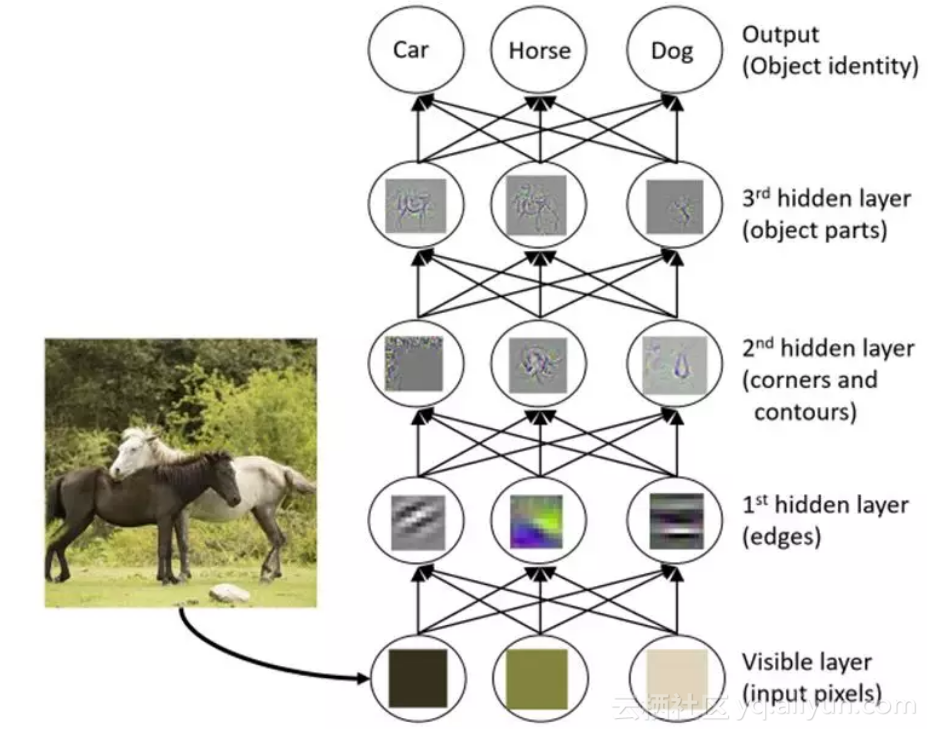●  layer-by-layer processing
●  in_model feature transformation

●  suffcient model complexity

(2) 集成学习中的灵感来源

●  数据样本机制： 它通过产生不同的数据样本来训练子模型；
●  输入特征机制：它通过选取不同特征子空间来训练子模型；
●  学习参数机制：他通过设定不同参数来使得子模型具有多样性；
●  输出表示机制：通过不同的输出表示增加子模型的多样性；

3.gcForest模型

(1) 级联森林结构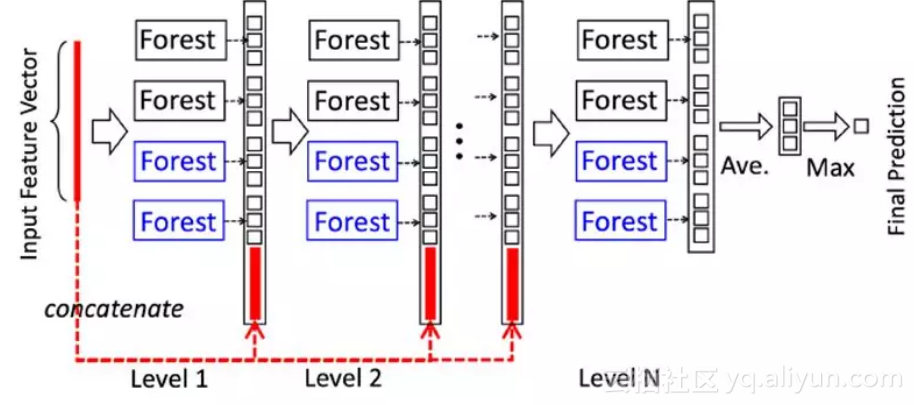●  每一个Level包含若干个集成学习的分类器(这里是决策树森林，你可以换成XGBoost,lightGBM等),这是一种集成中的集成的结构；
●  为了体现多样性，黑色和蓝色的Forest代表了若干不同的集成学习器，为了说明这里用了两种，黑色的是完全随机森林：由500棵决策树组成，每棵树随机选取一个特征作为分裂树的分裂节点，然后一直生成长，直到每个叶节点细分到只有一个类或者不多于10个样本，蓝色的表示普通的随机森林：由500棵决策树组成，每棵树通过随机选取sqrt(d)(d表示输入特征的维度)个候选特征然后通过gini系数筛选分裂节点；
●  gcForest采用了DNN中的layer-by-layer结构，从前一层输入的数据和输出结果数据做concat作为下一层的输入；
●  为了防止过拟合的情况出现，每个森林的训练都使用了k-折交叉验证，也即每一个训练样本在Forest中都被使用k-1次，产生k-1个类别列表，取平均作为下一个Level级联的输入的一部分；
●  当级联扩展到新的Level后，之前所有级联结构的表现通过验证集去评估，如果评估结果没有太大的改变或提升则训练过程结束，因此级联结构的Level的个数被训练过程决定；
●  这里要注注意的是，当我们想控制训练过程的loss或限制计算资源的时候，我们也可以使用训练误差，而不是交叉验证的误差同样能够用于控制级联的增长；

●  以上这一点就是gcForest和神经网络的区别，gcForest可以通过训练自适应的调整模型的复杂度，并且在恰当的时候停止增加我们的Level;

(2) 多粒度扫描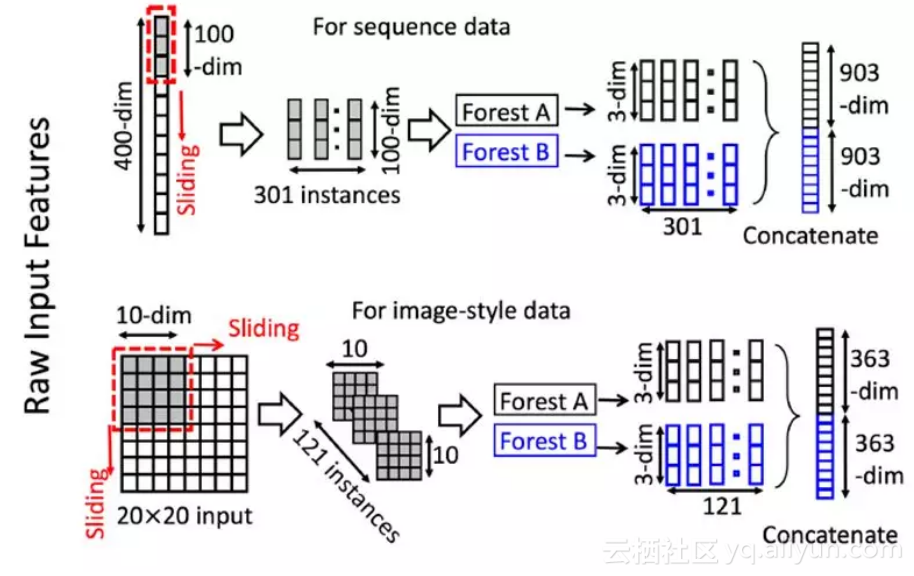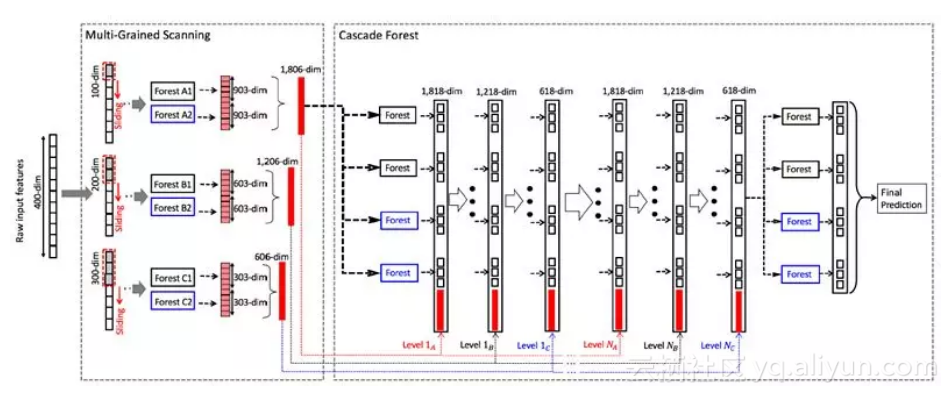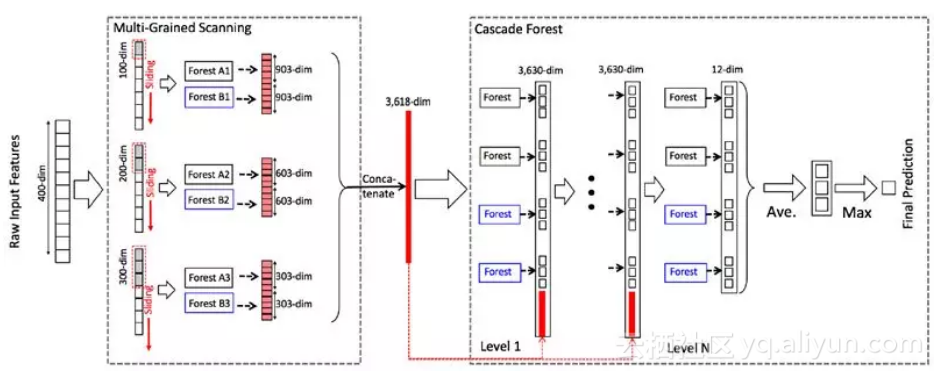(3) 小结

●  级联的每层的森林数
●  每个森林的决策树的数量
●  树停止生长的规则

●  多粒度扫描的森林数
●  每个森林的决策树数
●  树的停止生长规则
●  滑动窗口的数量和大小

●  计算开销小，单机就相当于DNN中使用GPU(如果你没有钱购买GPU,可以考虑使用gcForest)
●  模型效果好(周老师的papar中实验对比了不同模型的效果，gcForest在传统的机器学习，图像，文本，语音上都表现不俗)
●  超参数少，模型对超参数不敏感，一套超参数可以用到不同的数据集
●  可以适用于不同大小的数据集，模型复杂度可自适用的伸缩
●  每个级联生成使用交叉验证，避免过拟合

●  相对于DNN，gcForest更容易进行理论分析

4.gcForest模型的Python实现

GitHub上有两个star比较多的gcForest项目,在参考文献中我们已经列出来了，下面我们就使用这两个gcForest的Python模块去尝试使用gcForest模型去解决一些问题，这里要说明的是其中参考文献3是官方提供(由gcForest的作者之一Ji Feng维护)的一个Python版本。目前gcForest并没有相对好用的R语言接口，我们在近期将会推出R语言的gcForest包，敬请期待。这里我们仅介绍参考文献4中的gcForest模块，如果感兴趣，可以自行研究官方提供的gcForest模块(官方模块，集成模型的选择除了随机森林外还可以选择其他的结构像XGBoost,ExtraTreesClassifierLogisticRegression,SGDClassifier等，因此建议使用该模块)。这里就介绍参考文献4中gcForest的模块使用。

Example1: iris数据集预测

from GCForest import gcForest
from sklearn.model_selection import train_test_split
from sklearn.metrics import accuracy_score

import pandas as pd
import numpy as np

X = iris.data
y = iris.target
X_tr, X_te, y_tr, y_te = train_test_split(X, y, test_size=0.33)

# 注意里边的参数设置，详细的参数意义
# 可以参考源码中的参数解释
gcf = gcForest(shape_1X=4, window=2, tolerance=0.0)
gcf.fit(X_tr, y_tr)

# 类别预测
pred_X = gcf.predict(X_te)
print(pred_X)

pred_X_prob = gcf.predict_proba(X_te)
print(pred_X_prob)

# 模型评估
accuracy = accuracy_score(y_true=y_te, y_pred=pred_X)
print('gcForest accuracy : {}'.format(accuracy))

# 模型保存
from sklearn.externals import joblib
joblib.dump(gcf, 'my_iris_model.sav')

# 模型加载

Example2:Digits数据集预测

from GCForest import gcForest
from sklearn.model_selection import train_test_split
from sklearn.metrics import accuracy_score

import pandas as pd
import numpy as np

X = digits.data
y = digits.target
X_tr, X_te, y_tr, y_te = train_test_split(X, y, test_size=0.4)

# 注意参数设定
gcf = gcForest(shape_1X=[8,8], window=[4,6], tolerance=0.0, min_samples_mgs=10, min_samples_cascade=7)
gcf.fit(X_tr, y_tr)

# 类别预测
pred_X = gcf.predict(X_te)
print(pred_X)

# 模型评估
accuracy = accuracy_score(y_true=y_te, y_pred=pred_X)
print('gcForest accuracy : {}'.format(accuracy))

Example3:多粒度扫描和级联结构分离

# 模型根据y_tr进行训练
gcf = gcForest(shape_1X=[8,8], window=5, min_samples_mgs=10, min_samples_cascade=7)
X_tr_mgs = gcf.mg_scanning(X_tr, y_tr)

# 回调最后训练的随机森林模型
X_te_mgs = gcf.mg_scanning(X_te)

# 使用多粒度扫描的输出作为级联结构的输入，这里要注意
# 级联结构不能直接返回预测，而是最后一层级联Level的结果
# 因此，需要求平均并且取做大作为预测值

tmp = np.mean(pred_proba, axis=0)
preds = np.argmax(tmp, axis=1)
accuracy_score(y_true=y_te, y_pred=preds)

Example4:去除多粒度扫描的级联结构

tmp = np.mean(pred_proba, axis=0)
preds = np.argmax(tmp, axis=1)
accuracy_score(y_true=y_te, y_pred=preds)

5.小结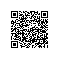Python爱好者使用钉钉扫一扫加入圈子
+ 订阅